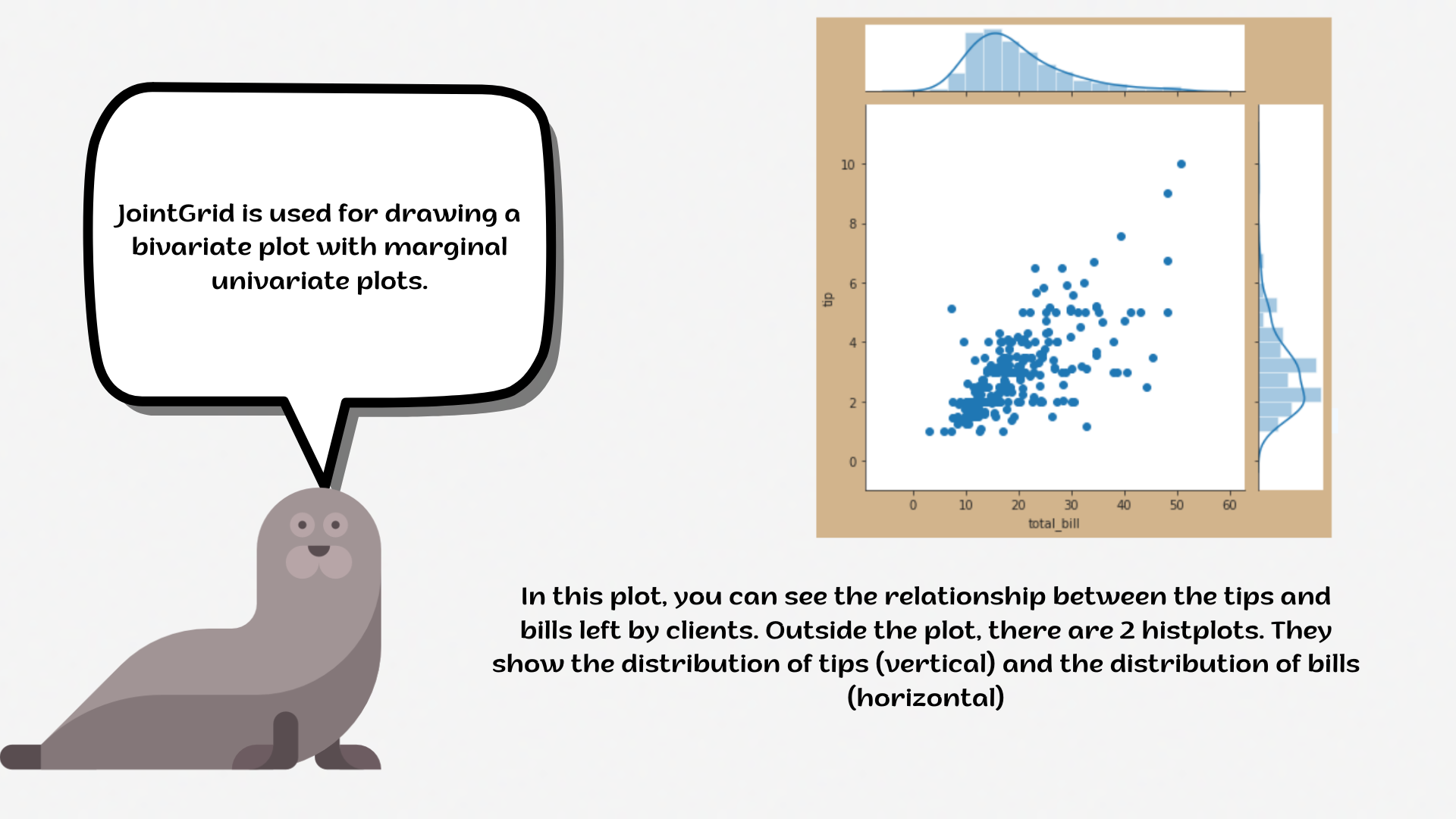Course Content

# Deep Dive into the seaborn Visualization

1. Light Start

2. Distributions of Data

3. Categorical Plot Types

4. Matrix Plots

5. Multi-Plot Grids

6. Regression Models

Deep Dive into the seaborn Visualization

##JointGrid`JointGrid` is a figure-level function, when the function is called a JointGrid object is instantiated. The function creates a JointGrid object consisting of three axes objects but does not plot anything on it.1. Set the `'ticks'` style with the `'lightcyan'` `figure.facecolor`.
2. Create a `JointGrid` variable `g`:
• Set the `x` parameter equals the `'bill_length_mm'`;
• Set the `y` parameter equals the `'bill_depth_mm'`;
• Set the `hue` parameter equals the `'species'`;
• Set the `'viridis'` palette;
• Set the data.

Set the inside plot using the `.plot_joint()` function using the `g` variable:

• Create a `scatterplot` using the `seaborn`;
• Set the `alpha` parameter equals `0.5`;
• Set the `'pink'` `edgecolor` parameter;
• Set the `linewidth` parameter equals `1`.

Set the outside plot using the `.plot_marginals()` function:

• Create the `histplot` using the `seaborn`;
• Add the `kde` parameter.

Everything was clear?

Section 5. Chapter 3